# Core Java. Lecture #2

## Demo

• Hello, World!

• New Maven Project

• New package

• New class

• psvm — sout

• Manifest (runnable jar)

## Language keywords [JLS17, 3.9]

``````abstract   continue   for          new         switch
assert     default    if           package     synchronized
boolean    do         goto         private     this
break      double     implements   protected   throw
byte       else       import       public      throws
case       enum       instanceof   return      transient
catch      extends    int          short       try
char       final      interface    static      void
class      finally    long         strictfp    volatile
const      float      native       super       while
_ (underscore)``````

## Null and Boolean Literals

``             null       true        false``

## Restricted Keywords & Identifiers

``````(Java 9)
open     module      requires    transitive    exports
opens    to          uses        provides      with

(Java 10)
var

(Java 14)
yield    record

(Java 15)
sealed   non-sealed  permits``````

``````/**
* This is the first sample program in Core Java Chapter 3
* @version 1.01 1997-03-22
* @author Gary Cornell
*/
public class FirstSample
{
public static void main(String[] args)
{
/*multiline
comment*/
System
.out //single-line comment
.println("We will not use 'Hello, World!'");
}
}``````

## Types

• Value

• Primitive

• `byte`, `short`, `int`, `long`

• `char`

• `float`, `double`,

• `boolean`

• Reference

• Arrays

• Objects

• `null`, damn it!

## Integer types

 Type Storage Range `byte` 1 byte –128 to 127 `short` 2 bytes –32,768 to 32,767 `int` 4 bytes –2,147,483,648 to 2,147,483, 647 `long` 8 bytes –9,223,372,036,854,775,808 to 9,223,372,036,854,775,807

## Varieties of integer literals

• Long `100L`

• Underscores `1_000_000`

• Hexadecimal `0xCAFEBABE`

• Binary `0b0101`

• Octal `010`

## Floating point types

 Type Storage Range `float` 4 bytes Approximately ±3.40282347E+38F (6–7 significant decimal digits) `double` 8 bytes Approximately ±1.79769313486231570E+308 (15 significant decimal digits)

## Literals for floating-point types

• `2.998e8`

• `1.0` (`1.`)

• `3.14F`

• `0x1.0p-3` (0.125, 2 to the power of minus three)

• `Double.POSITIVE_INFINITY`

• `Double.NEGATIVE_INFINITY`

• `Double.NaN`

## Be careful with floating point!

• `System.out.println(2.0 - 1.1)` outputs 0.8999999999999999

• NEVER USE DOUBLE FOR COUNTING MONEY!

• `System.out.println(2.0 / 0.0)` outputs 'Infinity` (but 2 / 0 is 'divizion by zero')

• `System.out.println(0.0 / 0.0)` outputs 'NaN`

• Comparison with infinity and `NaN` does not work, we need to use `Double.isNaN`, `Double.isInfinite`.

• `strictfp`

## Numeric types compatibility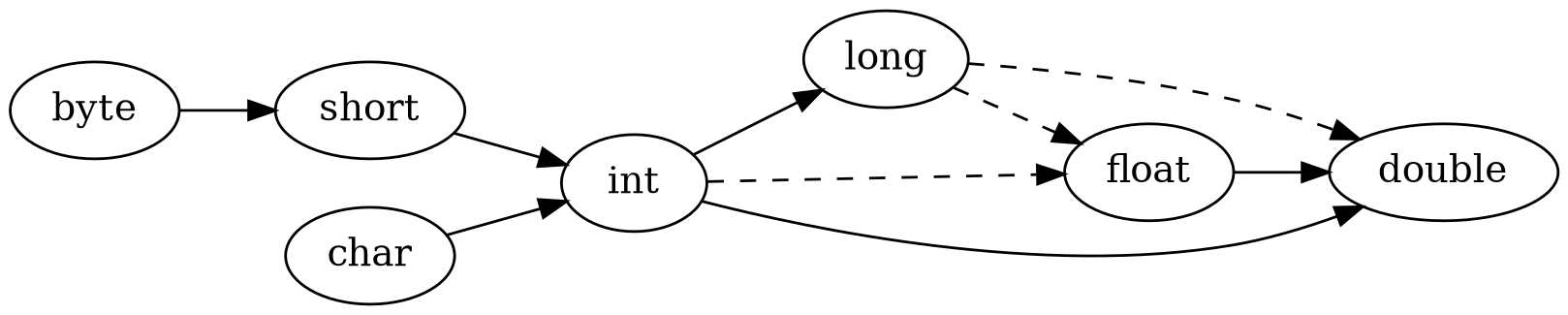## Explicit type casting

``````double x = 9.997F; //float to double converted implicitly
int nx = (int) x; //9
nx = (int) Math.round(x); //10``````

## `char` type

• 16 bit

• code unit in the UTF-16 encoding

• Not always a whole character (although almost always)!

• Be careful with Unicode, use 'String'

## `char` literals

• `'ы'`

• `'\u03C0'` (Greek π)

• `'\''` (escaped single quote)

• Unicode escape trap: `// Look inside c:\users`

## String literals

``````String s = "Hello, world!";

//Before Java 13
String txt =
"Some\n" +
"  Nested\n" +
"    Text\n" +
"  Is\n" +
"Here\n";``````

``````Some
Nested
Text
Is
Here``````

## Text Blocks (Java 13+)

``````    String txt = """
Some
Nested
Text
Is
Here
""";``````

``````//The maximum number of spaces ahead has been removed:
Some
Nested
Text
Is
Here``````

## Escape sequences

 Escape sequence Name Unicode Value `\b` Backspace \u0008 `\t` Tab \u0009 `\n` Linefeed \u000a `\r` Carriage return \u000d `\"` Double quote \u0022 `\'` Single quote \u0027 `\\` Backslash \u005c `\s` intentional whitespace (Java 13+) `\` line continuation (Java 13+)

## `boolean` type

• `true` and `false`

• Unlike C and many other languages, integers are not automatically cast to `boolean`

• Avoids `if (x = 0) {…` errors

## Variables declaration and scope

``````// declaration
double salary;
int vacationDays;
long earthPopulation;
boolean done;

// not very welcomed
int i, j;

// technically possible, but . . .
int суммаНДФЛ;``````

## Variables initialization

``````int vacationDays;
System.out.println(vacationDays); //COMPILATION ERROR
// variable not initialized``````

Possible initialization methods:

``````int vacationDays;
vacationDays = 12;``````
``int vacationDays = 12;``

## `final` keyword (constants)

• `final` is used in two cases:

• prohibits changing the value

• prevents overriding of methods/classes

``````final int a;
...
a = 42; // initialization

...
a = 43; // compilation error:
// variable a might already have been initialized``````

## Variable scope

• Place of definition: class, method, block

• In Java, there is nothing outside classes ("global" variables)!

``````int n;
. . .
{
int k;
int n; // Error--can't redefine n in inner block
. . .
} // k is only defined up to here``````

## Type inference when defining variables (Java 10+)

``var i = 1; // i is of int type``

## Type inference

``````//BEFORE JAVA 10
URL codefx = new URL("http://codefx.org");
URLConnection connection = codefx.openConnection();
``````//AFTER JAVA 10
var codefx = new URL("http://codefx.org");
var connection = codefx.openConnection();

## Arithmetic operators

• Arithmetic: `+`, `-`,`*`, `/`, `%`

• Division works as integer if both arguments are integer

• Unary `+` and `-`.

## Shifts

 `5` `0000 …​ 0000 0101` `5 << 1 == 10` `0000 …​ 0000 1010` `5 >> 1 == 2` `0000 …​ 0000 0010` `-5` `1111 …​ 1111 1011` `-5 << 1 == -10` `1111 …​ 1111 0110` `-5 >> 1 == -3` `1111 …​ 1111 1101` the highest (sign) bit is retained `-5 >>> 1 == 2147483645` `0111 …​ 1111 1101` the highest (sign) bit is filled with zero

## Don’t overdo it with shifts!!

`5 >> 32 == ?`

## Don’t overdo it with shifts!!

`5 >> 32 == 5`

• only 5 low-order bits of the second operand (0..31) are used for `int`,

• only 6 low-order bits of the second operand (0..63) are used for `long`

## Bitwise operators

• `&`, `|`, `^`, `~`

## Comparison operators

• `<` `<=` `>` `>=` `instanceof`

• `==` `!=`

## Boolean operators

• Without short circuiting: '&', '|', '^', '!' (exclamation instead of a tilde)

• With short-circuiting '&&', '|| `.

``x != 0 && 1 / x > x + y // no division by 0``

## Assignment operator

``````int x;
System.out.println(x = 42); //prints 42``````

Example of use:

``````while ((n = readValue()) != null)
... //do something with n``````

## Pre/post increment/decrement, assignment with change

• `a++`, `++a`

• `a--`, `--a`

• `+=`, `-=`, `*=`, `/=`, `%=`, `&=`, `|=`, `^=`, `<<=`, `>>=`, `>>>=`

• WARNING: These operations may appear to be atomic. But they are not.

## Ternary operator

``x < y ? x : y``

## Operators in descending order of priority

 Operators Associativity `[]` `.` `()` (method call) Left to right `!` `~` `++` `--` `+` (unary) `-` (unary) `()` (cast) `new` Right to left `*` `/` `%` Left to right `+` `-` Left to right `<<` `>>` `>>>` Left to right `<` `<=` `>` `>=` `instanceof` Left to right `==` `!=` Left to right

## Operators in descending order of priority (continued)

 Operators Associativity `&` Left to right `^` Left to right `|` Left to right `&&` Left to right `||` Left to right
``````//Conjunction operator has higher priority than disjunction, that's why
a || b && c
// is equivalent to
a || (b && c)``````

## Operators in descending order of priority (continued)

 Operators Associativity `? :` Right to left `=` `+=` `-=` `*=` `/=` `%=` `&=` `|=` `^=` `<<=` `>>=` `>>>=` Right to left
``````//Since += associates right to left, the expression
a += b += c
//means
a += (b += c)
//That is, the value of b += c
// (which is the value of b after the addition)

## What about the comma operator?

• There isn’t one!

• You can list multiple statements separated by commas in `for(…​)` and `try(…​)` blocks

## `if` statement

 ``````if (yourSales >= target) performance = "Satisfactory";``````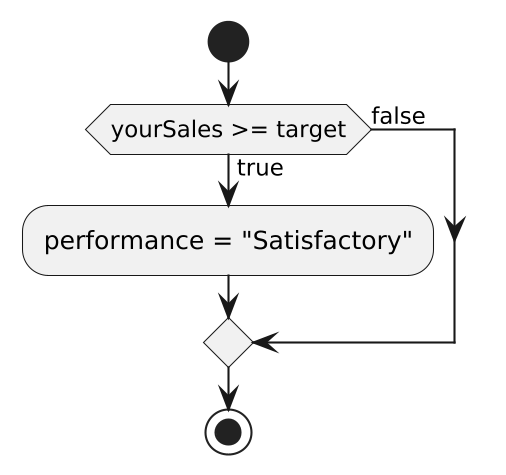## `if` + block

 ``````if (yourSales >= target) { performance = "Satisfactory"; bonus = 100; }``````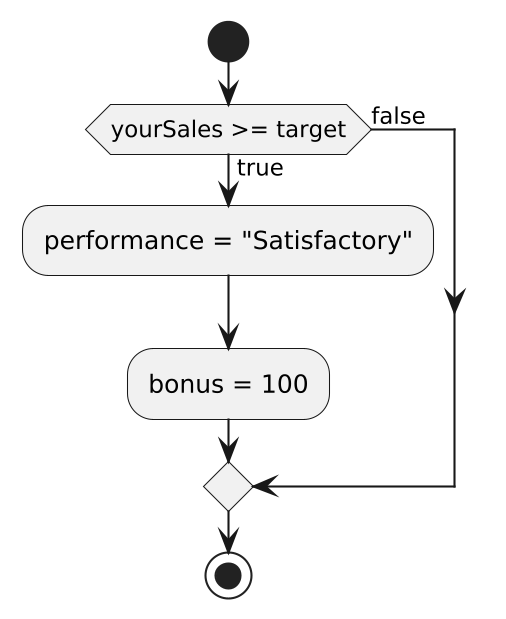## `if` + block + `else`

 ``````if (yourSales >= target) { performance = "Satisfactory"; bonus = 100 + 0.01 * (yourSales - target); } else { performance = "Unsatisfactory"; bonus = 0; }``````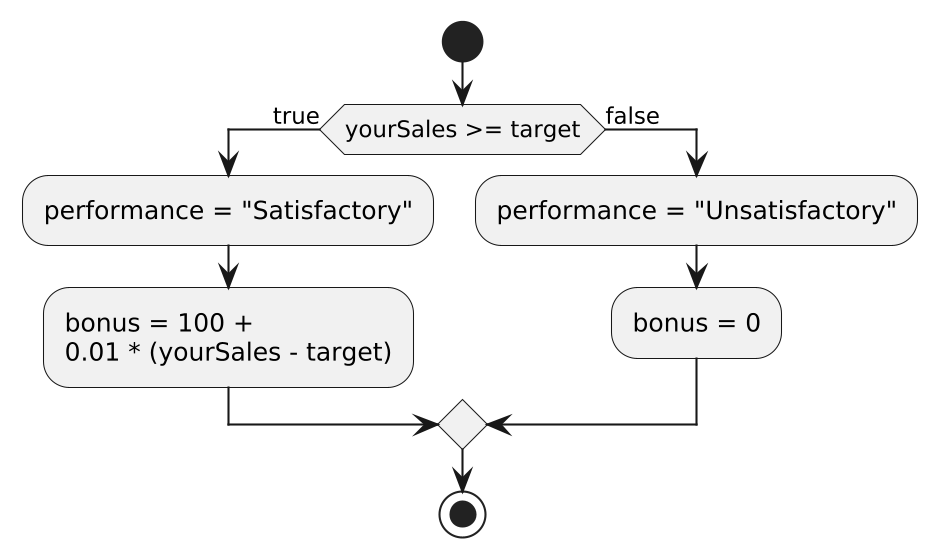## `else` is grouped with the nearest `if`

 ``````/* Better add curly braces!!*/ if (x <= 0) if (x == 0) sign = 0; else sign = -1;``````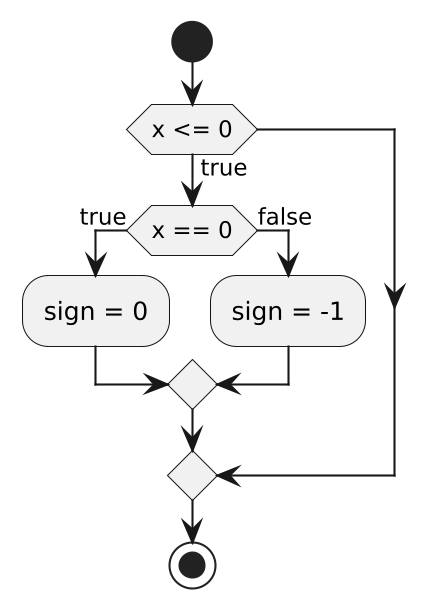## `else if` chains

 ``````if (sales >= 2 * target) { performance = "Excellent"; bonus = 1000; } else if (sales>=1.5*target) { performance = "Fine"; bonus = 500; } else if (sales >= target) { performance = "Satisfactory"; bonus = 100; } else { fireThisPerson(); }``````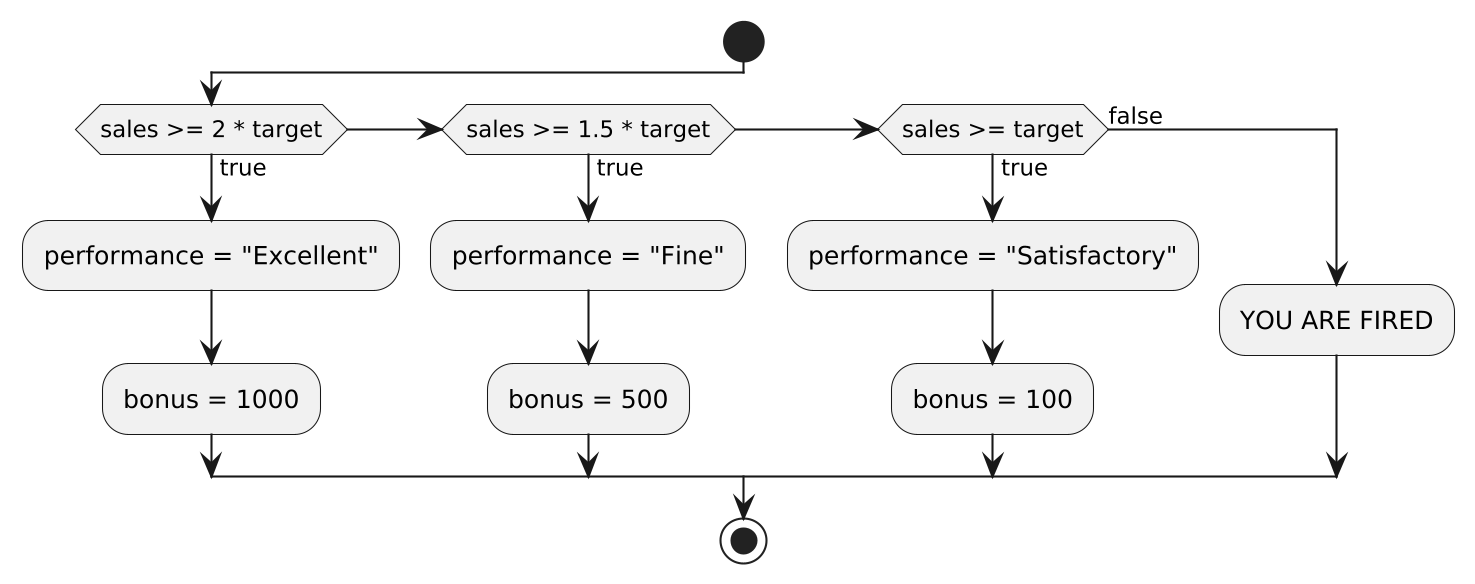## `switch`

Same nonsense as in C/C++:

 ``````switch (choice) { case 1: . . . break; case 2: . . . break; default: . . . break; }``````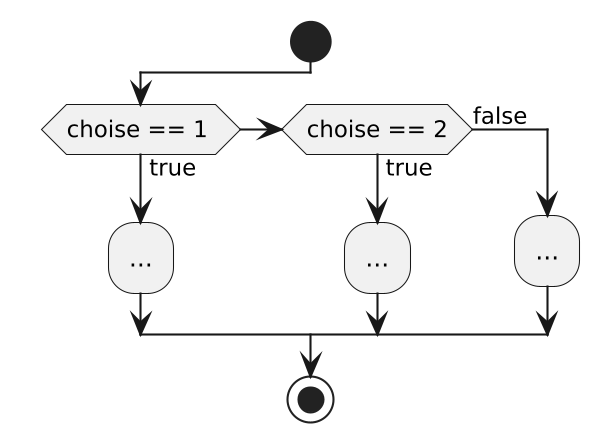## `switch`-`case` peculiarities:

• Do not forget about `break`s (Checkstyle-like utilities will remind you), or else all the code to the end of `switch` will be executed, like in C language!

• You can use `switch` with integers, `char`-s, `String`-s (Java 7+) and `enum`-s.

## Switch Expressions

• JDK 12 & 13 — Preview Feature

• JDK 14 — Standard Feature

``````int numLetters = switch(day) {
case MONDAY, FRIDAY, SUNDAY -> 6;
case TUESDAY -> 7;
case SATURDAY -> 8;
default -> 9;
}``````

## Switch in Java 14+: no Fallthrough

 Expression Statement ``````int numLetters = switch(day) { case MONDAY, FRIDAY, SUNDAY -> 6; case TUESDAY -> 7; case THURSDAY, SATURDAY -> 8; default -> 9; }`````` ``````switch(day) { case MONDAY, FRIDAY, SUNDAY -> numLetters = 6; case TUESDAY -> { logger.info("Tuesday"); numLetters = 7; } case THURSDAY, SATURDAY -> numLetters = 8; //Need not be exhaustive: //no WEDNESDAY }``````

## Switch in Java 14+: with Fallthrough

 Expression Statement (Good Old C-style Switch) ``````int numLetters = switch(day) { case MONDAY, FRIDAY, SUNDAY: yield 6; case TUESDAY: logger.info("Tuesday"); yield 7; case THURSDAY: logger.info("Thursday"); case SATURDAY: yield 8; default: yield 9; }`````` ``````switch(day) { case MONDAY, FRIDAY, SUNDAY: numLetters = 6; break; case TUESDAY: logger.info("Tuesday"); numLetters = 7; break; case THURSDAY: logger.info("Thursday"); case SATURDAY: numLetters = 8; break; default: numLetters = 9; }``````

## Arrays

• You can build an array of any type.

• The length of the array can be determined in the runtime, but cannot be changed after creation.

• The array is allocated on the heap and is passed by reference.

• Arrays verify the data type (`ArrayStoreException`) and boundaries ('ArrayIndexOutOfBoundsException') in the run time.

• Harsh Truth of Life: Chances are you won’t use arrays in modern code.

## Declaring and initializing an array

• Two options:

• `int[] a`

• 'int a[]' — don’t do that

• Initialization:

• `int[] a = new int;`

• `int[] a = {1, 3, 5};`

• anonymous array: `foo(new int[] {2, 4, 6});`

## Arrays are passed by reference

``````int[] luckyNumbers = smallPrimes;
luckyNumbers = 12; // now smallPrimes is also 12

luckyNumbers = Arrays.copyOf(luckyNumbers, 2 * luckyNumbers.length);
//now luckyNumbers is a separate array
//and it became twice as long``````

## Arrays are covariant

`````` String[] a = new String;
// Compiles. after all, a string is an object,
// and so why not think of an array of strings as an array of objects?
Object[] b = a;
// runtime ArrayStoreException
b = 5;``````

(All this is terribly incompatible with generics and Collections API that try to catch type errors at compile time, but more on that later.)

## "Multidimensional" arrays

There are none really, but use can build arrays of arrays.

 ``````int[][] magicSquare = { {16, 3, 2, 13}, {5, 10, 11, 8}, {9, 6, 7, 12}, {4, 15, 14, 1} }; /* magicSquare == ?? */``````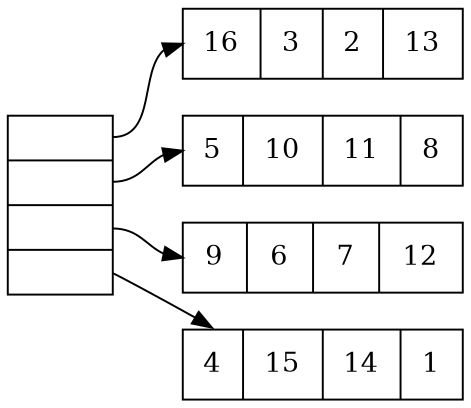## Triangular matrices

 ``````double[][] odds = new double[ROWNUM][]; for (int n = 0; n < ROWNUM; n++) odds[n] = new int[n + 1]; for (int n = 0; n < ROWNUM; n++) for (int k = 0; k <= n; k++){ /* compute lotteryOdds*/ . . . odds[n][k] = lotteryOdds; }``````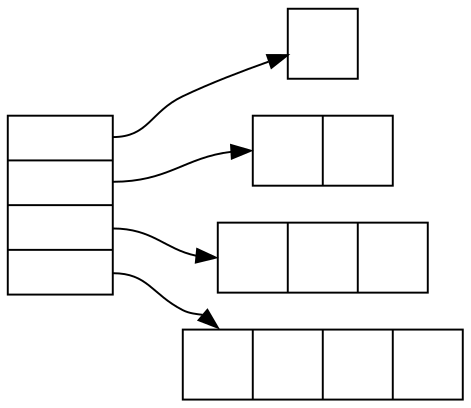## `while` loop: same as in C

 ``````while (balance < goal) { balance += payment; double interest = balance * interestRate / 100; balance += interest; years++; } System.out.println( years + " years.");``````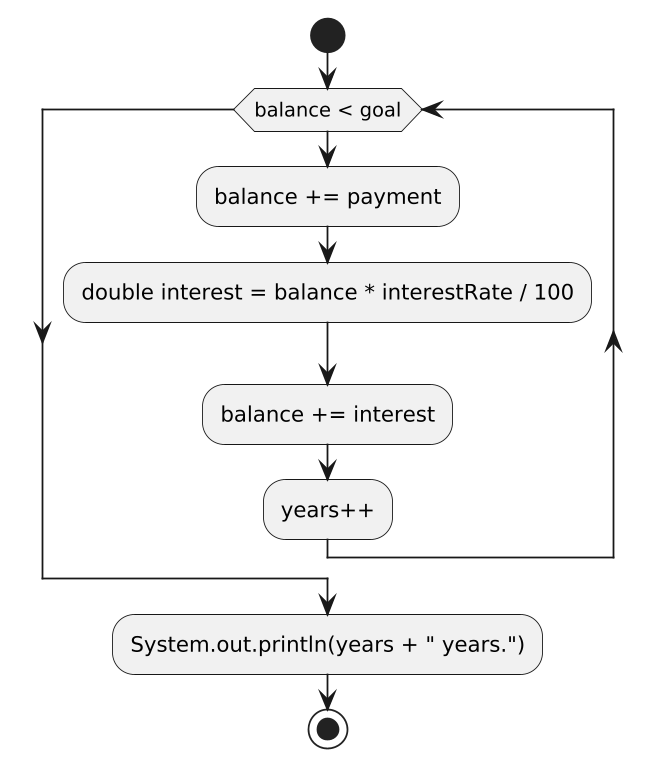## Same, but not the same!

``````final boolean flag = false;
. . .
while (flag) {
. . . //won't compile, unreachable code
}``````

## `do while`: loop with postcondition

 ``````do { balance += payment; double interest = balance * interestRate / 100; balance += interest; years++; // print current balance // ask if ready to retire and get input } while (input.equals("N"));``````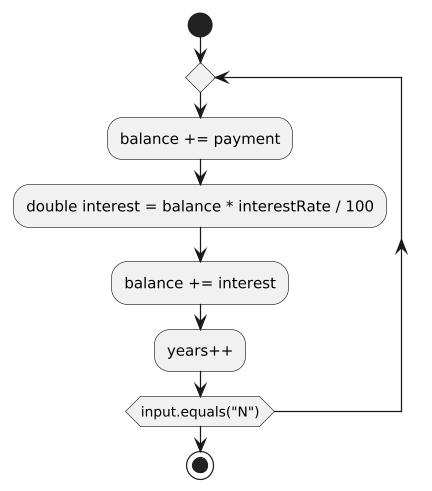Harsh Truth of Life: `do-while` is needed very rarely.

## `for` loops

Again, everything is the same as in C (in fact, the abbreviation of the `while` loop):

``````for (int i = 1; i <= 10; i++)
System.out.println(i);``````

And even though there is no "comma" operator, you can do this (but you don’t need to):

``````for (int i = 1; i <= 10; i++, j++)
System.out.println(i);``````

## Scope of the cycle variable

``````for (int i = 1; i <= 10; i++)
{
. . .
}
// i no longer defined here``````
``````int i;
for (i = 1; i <= 10; i++)
{
. . .
}
// i is still defined here``````

## Using `break` and `continue`

To interrupt the entire cycle:

``````while (years <= 100) {
balance += payment;
double interest = balance * interestRate / 100;
balance += interest;
if (balance >= goal) break;
years++;
}``````

Move on to the next cycle:

``````Scanner in = new Scanner(System.in);
while (sum < goal) {
System.out.print("Enter a number: ");
n = in.nextInt();
if (n < 0) continue;
sum += n; not executed if n < 0
}``````

## We don’t have `goto`, but there are labels for `break` and `continue`!

``````Scanner in = new Scanner(System.in);
int n;
// label is here!
read_data: while (. . .) {
. . .
for (. . .) {
System.out.print("Enter a number >= 0: ");
n = in.nextInt();
if (n < 0)